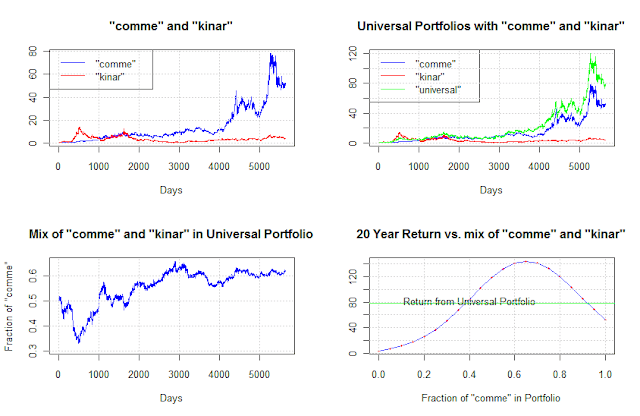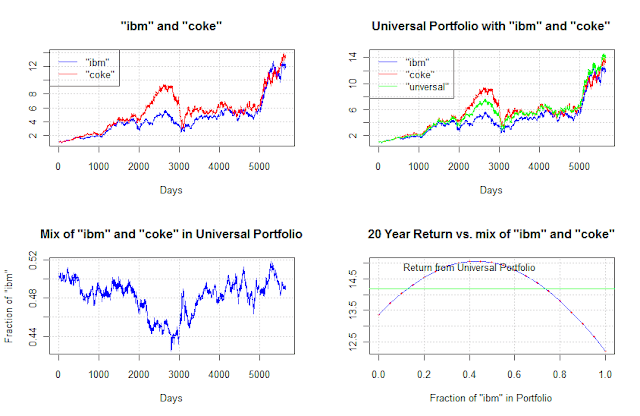## Thursday, June 7, 2012

### Universal portfolio, part 4

The graph for figure 8.4 requires to be somehow careful about correctly lagging the CRP value when calculating the weights.

# fig 8.4 of Cover "Universal Portfolios"
require(logopt)
x <- nyse.cover.1962.1984
xik <- x[,c("iroqu","kinar")]
nDays <- dim(xik)
Days <- 1:nDays
pik <- cumprod(xik)
alphas <- seq(0,1,by=0.05)
bk <- xik[,1] * 0
w <- xik[,1] * 0
for (i in 1:length(crps)) {
# we calculate bk by weighting the b by the realized wealth lagged one
weight <- lag(crp(xik, c(alphas[i], 1-alphas[i])), 1)
bk <- bk + alphas[i] * weight
w <- w + weight
}
bk <- bk / w
bk <- 0.5
plot(Days, bk, col="blue", type="l", ylim = range(0.25, range(bk)),
main = 'Mix of "iroqu" and "kinar" in Universal Portfolio',
ylab='Fraction of "iroqu"')
grid()The porfolio  b ^ k
Figure 8.5, 8.6 and 8.7 share the same format, so we define a function to draw them.

# fig 8.5, 8.6 and 8.7 of Cover "Universal Portfolios"

require(logopt)
x <- nyse.cover.1962.1984

show_pair <- function (x,a,b) {
xab <- x[,c(a,b)]
nDays <- dim(xab)
Days <- 1:nDays
pab <- cumprod(xab)
layout(matrix(c(1,3,4,2), 2, 2, byrow = TRUE))
main_text <- sprintf("\"%s\" and \"%s\"",a,b)
plot(Days, pab[,a], col="blue", type="l", ylim=range(pck),
main = main_text, ylab="")
lines(Days, pab[,b], col="red")
grid()
legend("topleft",c(sprintf("\"%s\"",a),
sprintf("\"%s\"",b)),col=c("blue","red"),lty=c(1,1))

alphas <- seq(0,1,by=0.05)
crps <- alphas
for (i in 1:length(crps)) {
crps[i] <- crp(xab, c(alphas[i], 1-alphas[i]))[nDays]
}
main_text <- sprintf("20 Year Return vs. mix of \"%s\" and \"%s\"",a,b)
xlab_text <- sprintf("Fraction of \"%s\" in Portfolio", a)
plot(alphas, crps, col="blue", type="l", ylab="",
main=main_text, xlab=xlab_text)
points(alphas, crps, pch=19, cex=0.5, col="red")
abline(h=mean(crps), col="green")
text(0.4,mean(crps)*1.05,labels="Return from Universal Portfolio")
grid()

universal <- xab[,1] * 0
for (i in 1:length(crps)) {
universal <- universal + crp(xab, c(alphas[i], 1-alphas[i]))
}
universal <- universal / length(alphas)
main_text <- sprintf("Universal Portfolio with \"%s\" and \"%s\"",a,b)
plot(Days, pab[,a], col="blue", type="l", ylim=range(pab, universal),
main = main_text, ylab="")
lines(Days, pab[,b], col="red")
lines(Days, universal, col="green",)
legend("topleft",c(sprintf("\"%s\"",a), sprintf("\"%s\"",b),'"unversal"'),
col=c("blue","red","green"),lty=c(1,1,1))
grid()

bk <- xab[,1] * 0
w <- xab[,1] * 0
for (i in 1:length(crps)) {
# we calculate bk by weighting the b by the realized wealth lagged one
weight <- lag(crp(xab, c(alphas[i], 1-alphas[i])), 1)
bk <- bk + alphas[i] * weight
w <- w + weight
}
bk <- bk / w
bk <- 0.5
main_text <- sprintf("Mix of \"%s\" and \"%s\" in Universal Portfolio",a,b)
ylab_text <- sprintf("Fraction of \"%s\"", a)
plot(Days, bk, col="blue", type="l", ylim = range(range(bk)),
main=main_text, ylab=ylab_text)
grid()
}

# fig 8.5
show_pair(x,"comme","kinar")Commercial Metals and Kin Ark
# fig 8.6
show_pair(x,"comme","kinar")Commercial Metals and Mei Corp.

# fig 8.7
show_pair(x,"coke","ibm")IBM and Coca-Cola
All original figures are now reproduced.

#### 1 comment:

1.hi, great to find your blog. While running this example I am facing problem with the following lines
bk <- bk + alphas[i] * weight
w <- w + weight

when + operation is executed, bk become null

Data:
numeric(0)

Index:
numeric(0)